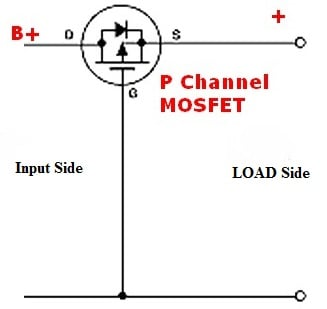# Diode replacement with MOSFET for Reverse Polarity Protection

3 posts / 0 newOffline

Joined: Sep 19, 2018

Replies: 0
Diode replacement with MOSFET for Reverse Polarity Protection
September 19, 2018 - 6:02pm

as i know Reverse polarity protection can be done only by using a simple diode, but i have checked few article and found that it is better to use a MOSFET instead of Diode.

Because voltage drop across diode is high. but i have tried the circuit with a general P-Channel MOSFET and after some calculation i found that the voltage drop is not much lower than the voltage across across diode..

i might be wrong, as i dont know how to choose the correct P-channel MOSFET,

of what parameter should be take care of...

Can anyone suggest me a best suitable P-Channel MOSFET for reverse polarity protection?

also, if it is possible with P-channel then i think its also possible with N-channel, what are the benefits of using these different methods...?

thanksOffline

Joined: Aug 16, 2016

Replies: 996

First off, which P-Channel MOSFET did you use?

The voltage across a P-Channel MOSFET should be less than a Diode. As you stated normally Schottky diods are used for Reverse polarity protection, but they are less efficient since they have a significant amount of voltage drop across them. We already had a similar discussion here

https://circuitdigest.com/forums/power-electronics/what-best-reverse-polarity-protection-circuit-battery

While using an P-Channel MOSFET the voltage drop should be less because of its low Drain to source resistance. I am posting a crude circuit below to explain why it is soYou have a lot of MOSFET options to select from, bt let us assume we are using the IRF9630 P-Channel MOSFET which according to the datasheet has only 0.08 ohm as Drain to Source resistance . Now assume that you have a 12V as you input Voltage and you load is connected to the load side. When the 12V is connected in correct polarity the MOSFET will conduct since the voltage drop across drain and gate VDG will be around -10.5V, considering 1.5V drop across the internal diode. This voltage will make the MOSFET conduct and thus power the load.

When the load is powered the current flows through Drain and Source pins of the MOSFET. If we assume a current of 1A through it then the voltage drop will be (V=IR) V = 1 * 0.08 whcih is only 0.08V.

In this same case if we have used a diode the drop would be a minimum of 0.2V, this a MOSFET is always efficient over a diode. But, still it has its own drawback like it cannot be used for low voltage applications since the value of RDS increase with decrease in voltage.

Yes you can also use a N-Channel MOSFET for protection but it will switch the negative line (low side switching) and hence it is not usually recommended.Offline

Joined: Nov 01, 2017

Replies: 3

While choosing a P-Channel MOSFET you have to check its drain-to-source resistance value. I have made a circuit using FQP47P06 P-Channel MOSFET whose drain to source resistance is 0.026 ohm which is very low..

https://circuitdigest.com/electronic-circuits/reverse-polarity-protectio...

let's assume that the value of current flowing through the transistor of your circuit is 1A..

then the power loss will be

Power loss = I2R

Power loss = (1)2 * 0.026

= 0.026W

and yes this value of voltage drop is not even considerable in high voltage circuit or even in low voltage circuit also